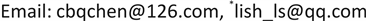﻿ 一类差分潘勒韦方程亚纯解的性质 Properties of Meromorphic Solutions of a Class of Difference Painlevé Equations

Advances in Applied Mathematics
Vol.07 No.07(2018), Article ID:25983,6 pages
10.12677/AAM.2018.77100

Properties of Meromorphic Solutions of a Class of Difference Painlevé Equations

Baoqin Chen, Sheng Li*

Faculty of Mathematics and Computer Science, Guangdong Ocean University, Zhanjiang GuangdongReceived: Jun. 23rd, 2018; accepted: Jul. 13th, 2018; published: Jul. 20th, 2018ABSTRACT

This paper studies a class of Painlevé difference equations of the form $w\left(z+1\right)w\left(z-1\right)=h\left(z\right){w}^{2}\left(z\right)$ where $h\left(z\right)\in S\left(w\right)$ is a rational function, and proves the following conclusions: 1) if w has finitely many zeros and poles, then $\rho \left(w\right)\in \left\{1,2\right\}$ and $\begin{array}{l}\begin{array}{l}w\hfill \end{array}\hfill \end{array}=R{e}^{{a}_{2}{z}^{2}+{a}_{1}z+{a}_{0}}$ , where R is a rational function, and ${a}_{0},{a}_{1},{a}_{2}$ are constants such that either ${a}_{1}\ne 0$ or ${a}_{2}\ne 0$ ; 2) if w has infinitely many zeros or poles, then $\rho \left(w\right)\ge max\left\{\lambda \left(w\right),\lambda \left(1/w\right)\right\}\ge 1$.

Keywords:Difference Painlevé Equations, Growth, Poles, ZerosCopyright © 2018 by authors and Hans Publishers Inc.

This work is licensed under the Creative Commons Attribution International License (CC BY).

http://creativecommons.org/licenses/by/4.0/1. 引言

$w\left(z+1\right)w\left(z-1\right)=R\left(z,f\right)$ (1)

$w\left(z+1\right)=\frac{\alpha \left(z\right)w\left(z\right)+\beta \left(z\right)}{w\left(z\right)+\gamma \left(z\right)},$

$w\left(z+1\right)w\left(z-1\right)=\frac{\eta \left(z\right){w}^{2}\left(z\right)-\lambda \left(z\right)w\left(z\right)+\mu \left(z\right)}{\left(w\left(z\right)-1\right)\left(w\left(z\right)-\nu \left(z\right)\right)},$ (2a)

$w\left(z+1\right)w\left(z-1\right)=\frac{\eta \left(z\right){w}^{2}\left(z\right)-\lambda \left(z\right)w\left(z\right)}{w\left(z\right)-1},$ (2b)

$w\left(z+1\right)w\left(z-1\right)=\frac{\eta \left(z\right)\left(w\left(z\right)-\lambda \left(z\right)\right)}{w\left(z\right)-1},$ (2c)

$w\left(z+1\right)w\left(z-1\right)=h\left(z\right){w}^{m}\left(z\right).$ (2d)

$\begin{array}{l}{\kappa }^{2}\left(z\right)\mu \left(z+1\right)\mu \left(z-1\right)={\mu }^{2}\left(z\right),\\ \lambda \left(z+1\right)\mu \left(z\right)=\kappa \left(z\right)\lambda \left(z-1\right)\mu \left(z+1\right),\\ \kappa \left(z\right)\lambda \left(z+2\right)\lambda \left(z-1\right)=\kappa \left(z-1\right)\lambda \left(z\right)\lambda \left(z+1\right),\end{array}$

1) $\eta \equiv 1$ ，且 $\lambda \left(z\right)=\lambda \left(z+1\right)\lambda \left(z-1\right)$$\lambda \left(z+3\right)\lambda \left(z-3\right)=\lambda \left(z+2\right)\lambda \left(z-2\right)$ 成立；

2) $\lambda \left(z+1\right)\lambda \left(z-1\right)=\lambda \left(z+2\right)\lambda \left(z-2\right),\text{\hspace{0.17em}}\eta \left(z+1\right)\lambda \left(z+1\right)=\lambda \left(z+2\right)\eta \left(z-1\right),\text{\hspace{0.17em}}\eta \left(z\right)\eta \left(z-1\right)=\eta \left(z+2\right)\eta \left(z+3\right)$

3) $\eta \left(z+2\right)\eta \left(z-2\right)=\eta \left(z\right)\eta \left(z-1\right),\text{\hspace{0.17em}}\lambda \left(z\right)=\eta \left(z-1\right)$

4) $\lambda \left(z+3\right)\lambda \left(z-3\right)=\lambda \left(z+2\right)\lambda \left(z-2\right)\lambda \left(z\right),\text{\hspace{0.17em}}\eta \left(z\right)\lambda \left(z\right)=\eta \left(z+2\right)\eta \left(z-2\right)$

$w\left(z+1\right)w\left(z-1\right)=h\left(z\right){w}^{2}\left(z\right),$ (3)

1) 若w只有有限个零点和极点，则 $\rho \left(w\right)\in \left\{1,2\right\}$$\begin{array}{l}\begin{array}{l}w\hfill \end{array}\hfill \end{array}=R{\text{e}}^{{a}_{2}{z}^{2}+{a}_{1}z+{a}_{0}}$ ，其中R为有理函数， $\begin{array}{l}{a}_{0}\hfill \end{array},{a}_{1},{a}_{2}$ 为常数， ${a}_{1},{a}_{2}$ 不同时为0；

2) 若w有无穷多个零点或极点，则 

$w\left(z+1\right)w\left(z-1\right)=\frac{{z}^{2}-1}{{z}^{2}}{w}^{2}\left(z\right)$

$\rho \left({\begin{array}{l}w\hfill \end{array}}_{1}\right)=+\infty ,\text{\hspace{0.17em}}\rho \left({\begin{array}{l}w\hfill \end{array}}_{2}\right)=\rho \left({\begin{array}{l}w\hfill \end{array}}_{3}\right)=\rho \left({\begin{array}{l}w\hfill \end{array}}_{4}\right)=\rho \left({\begin{array}{l}w\hfill \end{array}}_{5}\right)={\rho }_{2}\left({\begin{array}{l}w\hfill \end{array}}_{1}\right)=1;\text{\hspace{0.17em}}\lambda \left(1/{w}_{4}\right)=\rho \left({\begin{array}{l}w\hfill \end{array}}_{4}\right);\text{\hspace{0.17em}}\lambda \left({\begin{array}{l}w\hfill \end{array}}_{5}\right)=\rho \left({\begin{array}{l}w\hfill \end{array}}_{5}\right).$

2. 引理

$\begin{array}{l}f\left(z\right)={c}_{k}{z}^{k}+{c}_{k+1}{z}^{k+1}+\cdots ,\text{ }\hfill \end{array}{c}_{k}\ne 0,k\in ℤ,$

$f\left(z\right)={z}^{k}{\text{e}}^{Q\left(z\right)}\frac{{P}_{1}\left(z\right)}{{P}_{2}\left(z\right)},$

$h\left(z\right)\equiv \underset{z\to \infty }{\mathrm{lim}}\frac{w\left(z+1\right)w\left(z-1\right)}{{w}^{2}\left(z\right)}=1.$

$w\left(z+1\right)w\left(z-1\right)={w}^{2}\left(z\right).$ (4)

1) 当 $2{k}_{2}-{l}_{1}=0$ 时，−3既不是 $w\left(z\right)$ 的零点也不是 $w\left(z\right)$ 的极点，类似情况1可得类似的矛盾。

2) 当 $2{k}_{2}-{l}_{1}\le -1$ 时，−3为 ${l}_{1}-2{k}_{2}$ 阶极点。再由 ${w}^{2}\left(-3\right)=w\left(-2\right)w\left(-4\right)$ ，可知−4为 $w\left(z\right)$$2{l}_{1}-3{k}_{2}$ 阶极点。依次类推， $-n\left(n=5,6,7,\cdots \right)$ 均为 $w\left(z\right)$$\left(n-2\right){l}_{1}-\left(n-1\right){k}_{2}$ 阶极点，这表明w有无穷多个极点，与w为有理函数矛盾。

3) 当 $2{k}_{2}-{l}_{1}\ge 1$ 时，−3为 $w\left(z\right)$$2{k}_{2}-{l}_{1}$ 阶零点。再由 ${w}^{2}\left(-3\right)=w\left(-2\right)w\left(-4\right)$ ，由(1)和(2)中的讨论可知−4是 $w\left(z\right)$ 的极点且阶为 $3{k}_{2}-2{l}_{1}$ 。依此类推可得， $-n\left(n=5,6,7,\cdots \right)$ 均为 $w\left(z\right)$$\left(n-1\right){k}_{2}-\left(n-2\right){l}_{1}$ 阶零点。这表明w有无穷多个零点，与w为有理函数矛盾。

3. 定理1的证明

$w\left(z\right)={z}^{k}{\text{e}}^{Q\left(z\right)}\frac{{P}_{1}\left(z\right)}{{P}_{2}\left(z\right)},$ (5)

$h\left(z\right)=\frac{{\left(z+1\right)}^{k}{\left(z-1\right)}^{k}}{{z}^{2k}}\frac{{P}_{2}^{2}\left(z\right){P}_{1}\left(z+1\right){P}_{1}\left(z-1\right)}{{P}_{1}{}^{2}\left(z\right){P}_{2}\left(z+1\right){P}_{2}\left(z-1\right)}{\text{e}}^{2Q\left(z\right)-Q\left(z+1\right)-Q\left(z-1\right)},$

${\text{e}}^{Q\left(z+1\right)+Q\left(z-1\right)-2Q\left(z\right)}=\frac{{\left(z+1\right)}^{k}{\left(z-1\right)}^{k}}{{z}^{2k}h\left(z\right)}\frac{{P}_{2}^{2}\left(z\right){P}_{1}\left(z+1\right){P}_{1}\left(z-1\right)}{{P}_{1}{}^{2}\left(z\right){P}_{2}\left(z+1\right){P}_{2}\left(z-1\right)}.$ (6)

$\begin{array}{l}\begin{array}{l}Q\left(z\right)\hfill \end{array}\hfill \end{array}={a}_{n}{z}^{n}+\cdots +{a}_{1}z+{a}_{0},$

$Q\left(z+1\right)+Q\left(z-1\right)-2Q\left(z\right)=c.$ (7)

1) 当 $n=1$ 时， $Q\left(z+1\right)+Q\left(z-1\right)-2Q\left(z\right)=0$

2) 当 $n=2$ 时， $Q\left(z+1\right)+Q\left(z-1\right)-2Q\left(z\right)=2{C}_{2}^{2-2}{a}_{2}{z}^{2-2}$

3) 当 $n=3$ 时， $Q\left(z+1\right)+Q\left(z-1\right)-2Q\left(z\right)=2\cdot \left({C}_{3}^{2}{a}_{3}{z}^{3-2}+{C}_{2}^{2}{a}_{2}{z}^{2-2}\right)$

$Q\left(z+1\right)+Q\left(z-1\right)-2Q\left(z\right)=2\sum _{j=2}^{n}{C}_{j}^{j-2}{a}_{j}{z}^{j-2}\triangleq {b}_{n-2}{z}^{n-2}+{b}_{n-3}{z}^{n-3}+\cdots +{b}_{0},$

$Q\left(z\right)={a}_{2}{z}^{2}+{a}_{1}z+{a}_{0},$

$\lambda \left(r,w\right)=\underset{r\to +\infty }{\mathrm{lim}}\mathrm{sup}\frac{\mathrm{log}N\left(r,1/w\right)}{\mathrm{log}r}\ge \underset{s\to +\infty }{\mathrm{lim}}\frac{\mathrm{log}\left(\left(s-2-k\right)\mathrm{log}s\right)}{\mathrm{log}\left(s+|{z}_{m}|\right)}=1,$

$\lambda \left(r,1/w\right)=\underset{r\to +\infty }{\mathrm{lim}}\mathrm{sup}\frac{\mathrm{log}N\left(r,w\right)}{\mathrm{log}r}\ge \underset{s\to +\infty }{\mathrm{lim}}\frac{\mathrm{log}\left(\left(s-2-k\right)\mathrm{log}s\right)}{\mathrm{log}\left(s+|{z}_{m}|\right)}=1.$

$\rho \left(w\right)\ge \mathrm{max}\left\{\lambda \left(w\right),\lambda \left(1/w\right)\right\}\ge \text{1}\text{.}$

Properties of Meromorphic Solutions of a Class of Difference Painlevé Equations[J]. 应用数学进展, 2018, 07(07): 836-841. https://doi.org/10.12677/AAM.2018.77100

1. 1. Laine, I. (1993) Nevanlinna Theory and Complex Differential Equations. W.de Gruyter, Berlin. https://doi.org/10.1515/9783110863147

2. 2. 杨乐. 值分布论及其新研究[M]. 北京: 科学出版社, 1982.

3. 3. 何育赞, 袁文俊, 李叶舟. 潘勒韦方程解析理论讲义[M]. 北京: 科学出版社, 2005.

4. 4. Ablowitz, M., Halburd, R.G. and Herbst, B. (2000) On the Extension of Painlevé Property to Difference Equations. Nonlinearity, 13, 889-905. https://doi.org/10.1088/0951-7715/13/3/321

5. 5. Halburd, R.G. and Korhonen, R. (2007) Finite-Order Meromorphic Solutions and the Discrete Painlevé Equations. Proceedings of the London Mathematical Society, 94, 443-474. https://doi.org/10.1112/plms/pdl012

6. 6. Chen, Z.X. and Shon, K.H. (2010) Value Distribution of Meromorphic Solutions of Certain Difference Painlevé Equations. Journal of Mathematical Analysis and Applications, 364, 556-566. https://doi.org/10.1016/j.jmaa.2009.10.021

7. 7. Ronkainen, O. (2010) Meromorphic Solutions of Difference Painlevé Equations. Ph.D. Thesis, Department of Physics and Mathematics, University of Eastern Finland, Joensuu.

8. 8. Lan, S.T. and Chen, Z.X. (2014) On Properties of Meromorphic Solutions of Certain Difference Painlevé Equations. Abstract and Applied Analysis, 2014, Article ID: 208701. https://doi.org/10.1155/2014/208701

9. 9. Lan, S.T. and Chen, Z.X. (2014) Zeros, Poles and Fixed Points of Meromorphic Solutions of Difference Painlevé Equations. Abstract and Applied Analysis, 2014, Article ID: 782024.

10. 10. Zhang, J.L. and Yi, H.X. (2013) Properties of Meromorphic Solutions of Painlevé III Difference Equations. Advances in Difference Equations, 2013, Article ID: 256. https://doi.org/10.1186/1687-1847-2013-256

11. 11. Zhang, J.L. and Yi, H.X. 2014 (2014) Borel Exceptional Values of Meromorphic Solutions of Painlevé III Difference Equations. Advances in Difference Equations, 2014, Article ID: 144.

12. 12. Halburd, R.G. and Korhonen, R. (2006) Difference Analogue of the Lemma on the Logarithmic Derivative with Ap-plications to Difference Equations. Journal of Mathematical Analysis and Applications, 314, 477-487. https://doi.org/10.1016/j.jmaa.2005.04.010

13. 13. Chiang, Y.M. and Feng, S.J. (2008) On the Nevanlinna Characteristic of f (z + η) and Difference Equations in the Complex Plane. Ramanujan Journal, 16, 105-129. https://doi.org/10.1007/s11139-007-9101-1

14. 14. Yang, C.C. and Yi, H.X. (2003) Uniqueness Theory of Meromorphic Functions. Kluwer Academic Publishers, The Netherlands. https://doi.org/10.1007/978-94-017-3626-8

15. NOTES

*通讯作者。